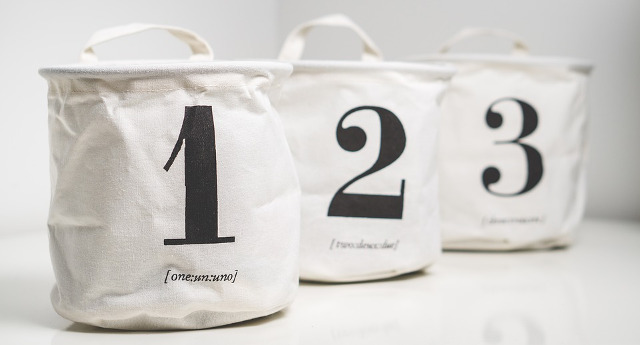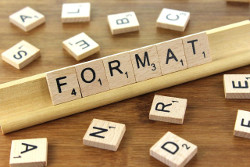# Convert between int, int64 and string

yourbasic.org/golang## int/int64 to string

Use `strconv.Itoa` to convert an int to a decimal string.

``s := strconv.Itoa(97) // s == "97"``
Warning: In a plain conversion the value is interpreted as a Unicode code point, and the resulting string will contain the character represented by that code point, encoded in UTF-8.
``````s := string(97) // s == "a"
``````

Use `strconv.FormatInt` to format an int64 in a given base.

``````var n int64 = 97
s := strconv.FormatInt(n, 10) // s == "97" (decimal)
``````
``````var n int64 = 97
s := strconv.FormatInt(n, 16) // s == "61" (hexadecimal)
``````

## string to int/int64

Use `strconv.Atoi` to parse a decimal string to an int.

``````s := "97"
if n, err := strconv.Atoi(s); err == nil {
fmt.Println(n+1)
} else {
fmt.Println(s, "is not an integer.")
}
// Output: 98``````

Use `strconv.ParseInt` to parse a decimal string (base `10`) and check if it fits into an int64.

``````s := "97"
n, err := strconv.ParseInt(s, 10, 64)
if err == nil {
fmt.Printf("%d of type %T", n, n)
}
// Output: 97 of type int64``````

The two numeric arguments represent a base (0, 2 to 36) and a bit size (0 to 64).

If the first argument is 0, the base is implied by the string’s prefix: base 16 for `"0x"`, base 8 for `"0"`, and base 10 otherwise.

The second argument specifies the integer type that the result must fit into. Bit sizes 0, 8, 16, 32, and 64 correspond to `int`, `int8`, `int16`, `int32`, and `int64`.

## int to int64 (and back)

The size of an `int` is implementation-specific, it’s either 32 or 64 bits, and hence you won’t lose any information when converting from int to  int64.

``````var n int = 97
m := int64(n) // safe
``````
However, when converting to a shorter integer type, the value is truncated to fit in the result type's size.
``````var m int64 = 2 << 32
n := int(m)    // truncated on machines with 32-bit ints
fmt.Println(n) // either 0 or 4,294,967,296
``````

## General formatting (width, indent, sign)

The `fmt.Sprintf` function is a useful general tool for converting data to string:

``````s := fmt.Sprintf("%+8d", 97)
// s == "     +97" (width 8, right justify, always show sign)``````

### Further readingSee this fmt cheat sheet for more about formatting integers and other types of data with the fmt package.